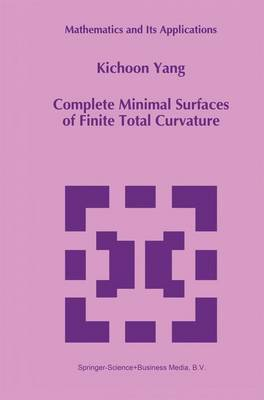•Complete Minimal Surfaces of Finite Total Curvature - Mathematics and Its Applications 294 (Hardback)

(author)
£109.99
Hardback 160 Pages / Published: 31/07/1994
• We can order this

Usually dispatched within 3 weeks

This monograph contains an exposition of the theory of minimal surfaces in Euclidean space, with an emphasis on complete minimal surfaces of finite total curvature. Our exposition is based upon the philosophy that the study of finite total curvature complete minimal surfaces in R3, in large measure, coincides with the study of meromorphic functions and linear series on compact Riemann sur- faces. This philosophy is first indicated in the fundamental theorem of Chern and Osserman: A complete minimal surface M immersed in R3 is of finite total curvature if and only if M with its induced conformal structure is conformally equivalent to a compact Riemann surface Mg punctured at a finite set E of points and the tangential Gauss map extends to a holomorphic map Mg _ P2. Thus a finite total curvature complete minimal surface in R3 gives rise to a plane algebraic curve. Let Mg denote a fixed but otherwise arbitrary compact Riemann surface of genus g. A positive integer r is called a puncture number for Mg if Mg can be conformally immersed into R3 as a complete finite total curvature minimal surface with exactly r punctures; the set of all puncture numbers for Mg is denoted by P (M ). For example, Jorge and Meeks [JM] showed, by constructing an example g for each r, that every positive integer r is a puncture number for the Riemann surface pl.

Publisher: Springer
ISBN: 9780792330127
Number of pages: 160
Weight: 930 g
Dimensions: 234 x 156 x 11 mm
Edition: 1994 ed.